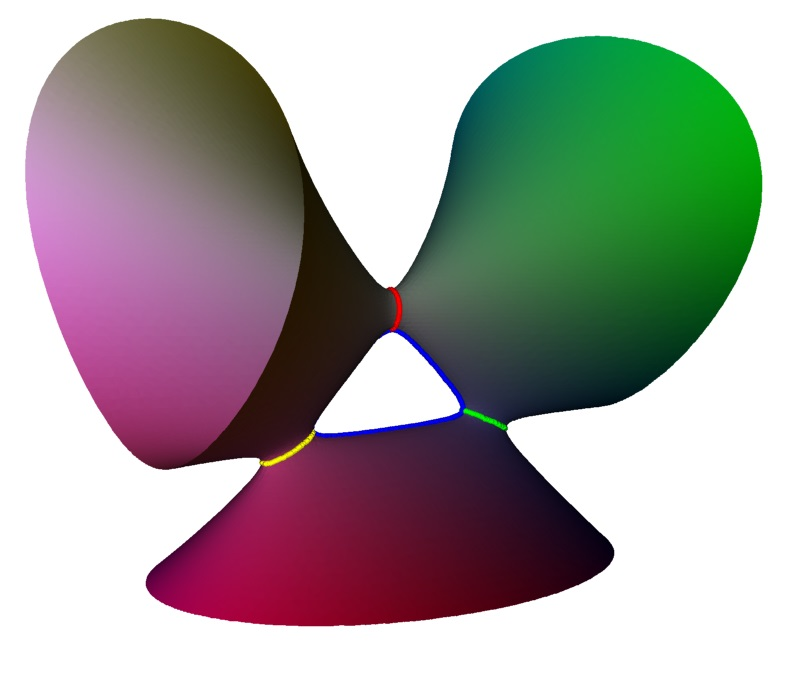Interactive apps

Here we will attempt to explain the connection between the classification of singularities and the ADE classification of Coxeter groups.

The best explanation I've found for this is Joris van Hoboken's Masters Thesis Platonic solids, binary polyhedral groups, Kleinian singularities and Lie algebras of type A,D,E (University of Amsterdam, 2002)

The basic idea is of blowing up singularities, this replaces each A1 singular point by a circle creating a smooth surface. Higher singularities have more complex blowups, A2 blows up to two linked circles, A3 to three circles, D4 to three circles all linked to a common fourth circle.

Calculating the homology group of this resulting surface allows the different types of singularity to be identified. These homology groups also link into the Coxeter Dynkin diagrams and the ADE classification.

Resolution of singularities

The goal of a resolution of a singularity is replacing a surface $V$ with singularity by another surface $U$ which is non-singular and away from the singular point is the same (isomorphic). To get a resolution we use a technique called a blow-up, this replaces the singular point by a line.

Consider this map from a cylinder to an A1 singularity.

The cylinder is defined as $x^2+y^2=1$ and the map $\mu: (x,y,z) \to (x z, y z,z)$ produces the A1 singular surface $x^2+y^2-z^2=0$. The circle $x^2+y^2=1, z=0$ is sent to the singular point and is called the exceptional set. Away from the circle the map is 1 to 1 and onto.

One particular feature of this map is if you take lines that cross the circle they map to lines that pass through the singularity. So we can thing of replacing the singularity by the set of lines passing through it.

Unfolding

A related concept is unfolding. Here we consider our singular surface as part of a family of surfaces, most of which are not singular. For the A1 singularity $x^2 + y^2 - z^2 =0$ we can consider the family $x^2 + y^2 - z^2 + d=0$ for different values of $d$.

We note here that one part of the unfolding gives a surface that is topologically the same as the blowup of the singularity. In particular both have one loop round the round the surface. In this hand-wavy explanation we blur the distinction between resolutions, blow-ups and unfoldings.

To represent these of the Coxeter-Dynkin diagram we can replace a solid circle with an open circle.Coxeter-Dynkin diagram for an A1 singularity with a single node (left), diagram representing the unfolding with one open circle representing the loop in the diagram (right).

Unfolding A2

The A2 singularity is represented by Coxeter-Dynkin diagram with two linked nodes.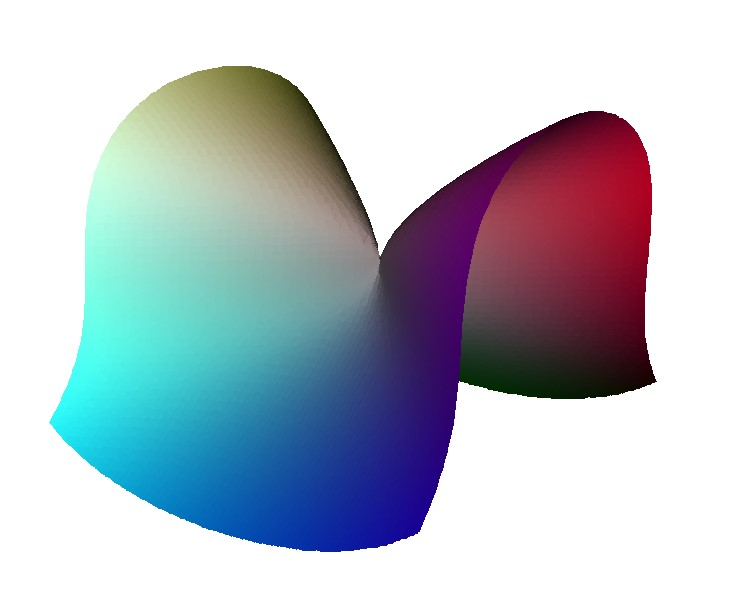3D Model: A2 singularity

To see how this comes about consider this unfolding of an A2 singularity. It happens in two stages, first a loop is created with an A1 point on it. Then this A1 point is unfolded to form a second loop.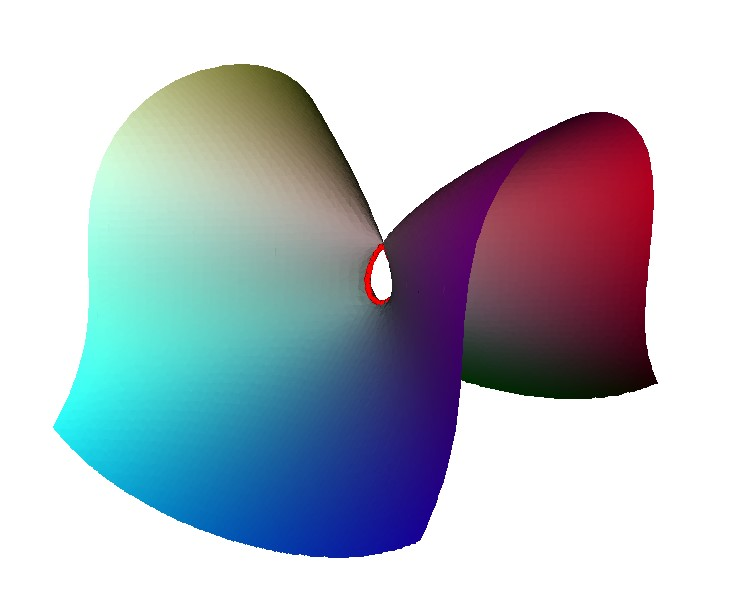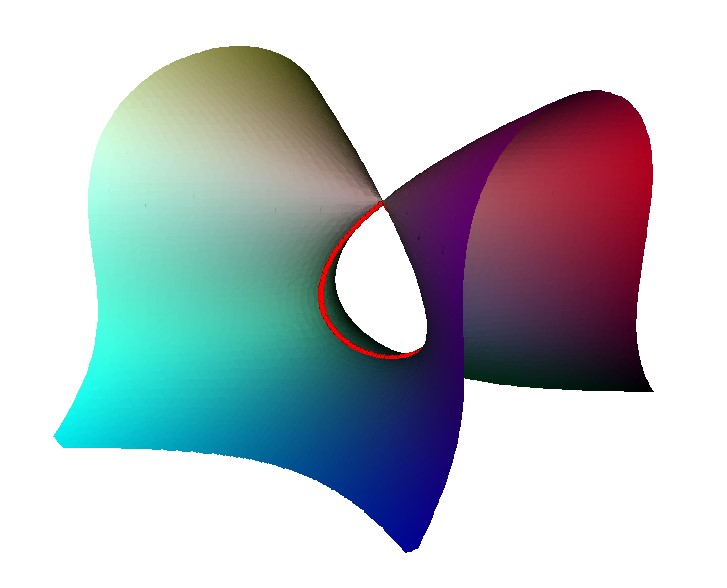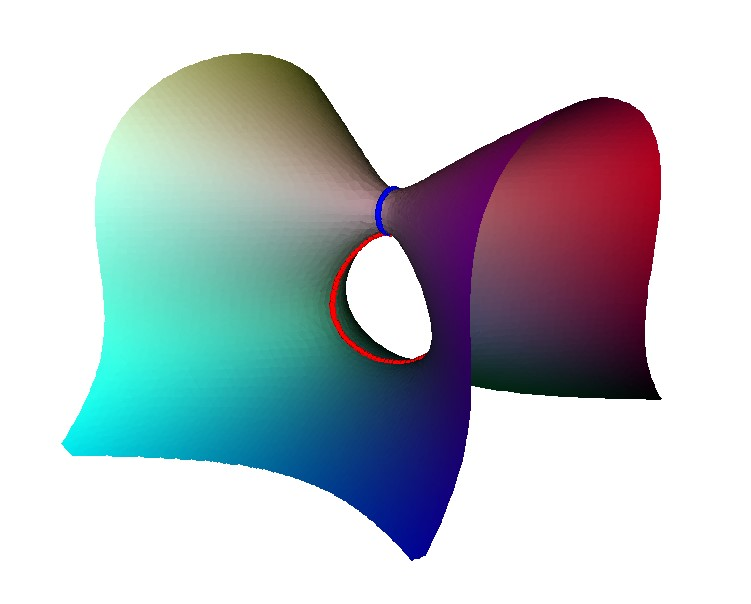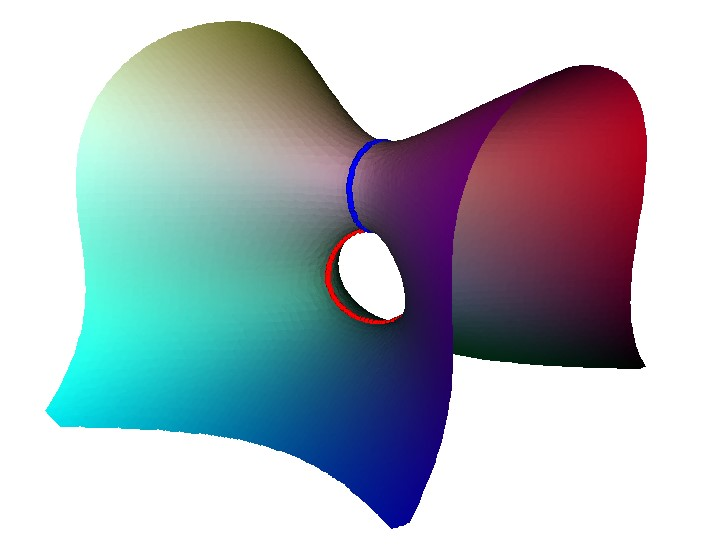The result of the unfolding give two linked loop. Each of these corresponds to a node on the Dynkin diagram, and as they are linked there is an edge in the Dynkin diagram.

3D Model: Unfold stage 1

3D Model: Unfold stage 2

For the A3 singularity there is similar pattern the singularity can be unfolded to three linked loops. There are various intermediate states with either an A2 singularity; two A1 singularities or a single A1 singularity.

The D4 singularity is the first one where the Coxeter-Dynkin diagram has a branch:.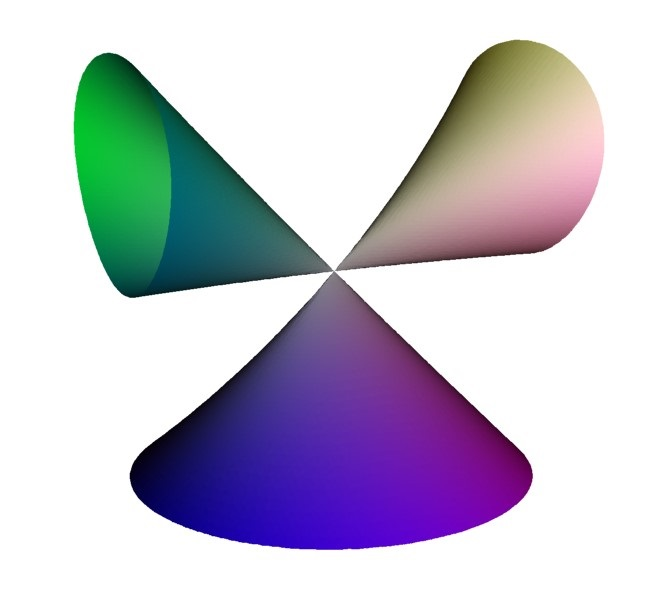3D Model: unfolded D4 singularity

The first stage of unfolding can occur in two different ways. One equivalent to removing the central node, giving rise to a shape with 3 A1 singularities. Alternatively one of the outer nodes can be removed, leaving an A3 singularity. Here we have used the unfolding $x^2 y - y^3 - z^2 + a ( y^2 + x y) + b y^2$, $a=b=0$ give the singularity changing the $s$ parameter gives the 3 A1 configuration going top to bottom, and change $b$ give the left-right configuration.